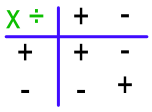Multiplying and Dividing Negative Numbers

# Multiplying and Dividing Negative Numbers

Basic, GCSE(F),

When multiplying and dividing negative numbers, the sign of both numbers is important:

+ multiplied by + gives a positive answer

- multiplied by - gives a positive answer

+ multiplied by - gives a negative answer

- multiplied by + gives a negative answer.

The same rules apply when dividing.

For these calculations, carry out the multiplication or division as if both numbers were positive, then apply the rule afterwards.

This table can be used as a reminder:## Examples

1. Evaluate -6 x -4.